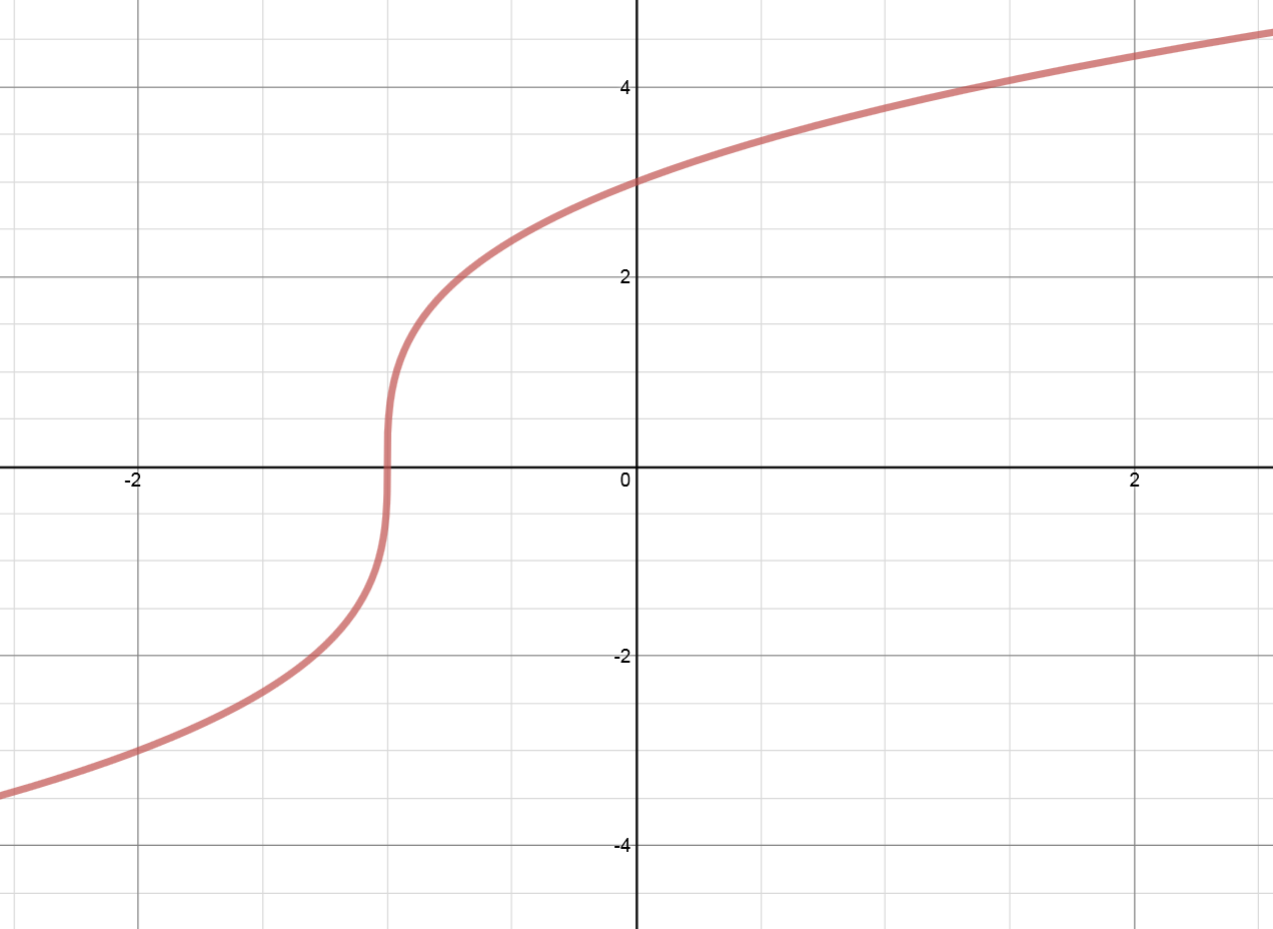# Transformations of functions: Vertical stretches - Relations and Functions

### Transformations of functions: Vertical stretches

#### Lessons

##### In conclusion: • $\left( y \right) \to \left( {2y} \right)$: vertical stretch by a factor of ________ ⇒ all $y$ coordinates ______________________. • $\left( y \right) \to \left( {\frac{3}{4}y} \right)$: vertical stretch by a factor of ________ ⇒ all $y$ coordinates ______________________.